Raja had an unbiased die with him and he challenged Radha, who had two unbiased dice with her. He said that he could produce a number on his die after rolling such that the number is greater than the sum of the numbers that Radha's dice produced. What is the probability that Raja's number is actually greater than the sum of the numbers of Radha's dice?
• a)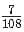• b)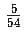• c)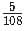• d)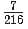### Related TestPathways Academy Jan 18, 2022The minimum number that Radha's die can show is 1 and 1, which sums up to 2.
So. Raja must have his dice show numbers from 3 to 6 including both,
When Raja's dice shows 3, Radha's die can show only (1,1). Probability of this happening=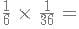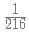When Raja's dice shows 4, Radha's die can show (1,1), (1,2) or (2,1). Probability of this happening=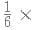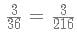When Raja's dice shows 5, Radha's die can show (1,1), (1,2), (2,1), (2,2), (1,3), (3,1). Probability of this happening=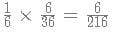When Raja's dice shows 6, Radha's die can show (1,1), (1,2), (2,1), (2,2), (1,3), (3,1), (3,2), (2,3), (4,1), (1,4). Probability of this happening=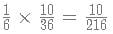.'. Required probability=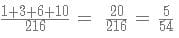The minimum number that Radhas die can show is 1 and 1, which sums up to 2.So. Raja must have his dice show numbers from 3 to 6 including both,When Rajas dice shows 3, Radhas die can show only (1,1). Probability of this happening=When Rajas dice shows 4, Radhas die can show (1,1), (1,2) or (2,1). Probability of this happening=When Rajas dice shows 5, Radhas die can show (1,1), (1,2), (2,1), (2,2), (1,3), (3,1). Probability of this happening=When Rajas dice shows 6, Radhas die can show (1,1), (1,2), (2,1), (2,2), (1,3), (3,1), (3,2), (2,3), (4,1), (1,4). Probability of this happening=.. Required probability=
Do you know? How Toppers prepare for CAT Exam
With help of the best CAT teachers & toppers, We have prepared a guide for student who are preparing for CAT : 15 Steps to clear CAT Exam